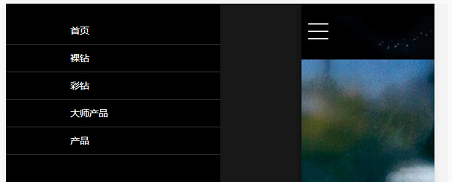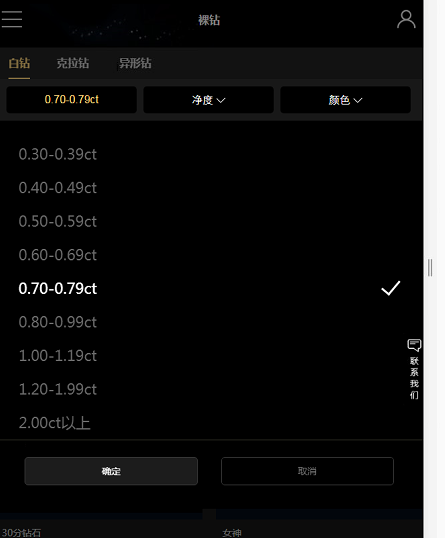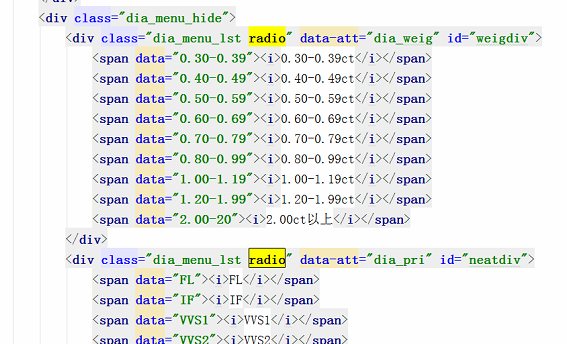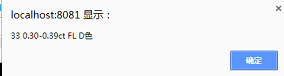HTML5前端页面取值问题// var weight=\$("[data-att='dia_weig']").val();
var ctgId = \$(".swiper-wrapper p").find("input").attr("value");
var weight =\$('#weigdiv span i').html();
var neatness =\$('#neatdiv span i').html();
var color =\$('#colordiv span i').html();

``````                        我的js取值代码
``````

7个回答

var data = \$(this).attr('data');
}2 年多之前 回复

1. var weight =\$('#weigdiv span i').html() 只能取 weigdiv span i 第一个元素，若想取选中，可用\$(this).find('i').html();
2. 当前选择方式只能选取点中div的span，无法选取其他div中的span ，需要做一个选中状态添加

2. 修改js代码如下
\$(this).siblings().each(function){
\$(this).removeClass("selected"); //去除同一个div下span兄弟节点的选中状态
}
\$(this).toogleClass("selected"); // 点击某个span时添加或移除选中状态

var ctgId = \$(".swiper-wrapper p").find("input").attr("value");
var weight =\$('#weigdiv .selected i').html(); //选择含有选中状态selected的元素（有选中状态状态的span）
var neatness =\$('#neatdiv .selected i').html();
var color =\$('#colordiv .selected i').html();
});2 年多之前 回复2 年多之前 回复2 年多之前 回复

`````` \$(".dia_menu_lst span").click(function (e) {
var ctgId = \$(this).find("input").attr("value");
var weight =\$(this).html();
var neatness =\$(this).html();
var color =\$(this).html();
``````

`````` function getValue(){
var ctgId = \$(".swiper-wrapper p").find("input").attr("value");
var weight =\$('#weigdiv span i').html();
var neatness =\$('#neatdiv span i').html();
var color =\$('#colordiv span i').html();
}
``````

``````// 第一个
\$("#wgidiv span").click(function (e) {
\$("#ajfds").val(this.data); // ajfds是个隐藏域
});

// 第二个
\$("#wwuiv span").click(function (e) {
\$("#wwuiv").val(this.data); // wwuiv是个隐藏域
})
``````

var weight =\$('#weigdiv span i').html();

\$('#weigdiv span i') 这样选取出来是一个集合，类似于数组，但不是数组。

\$('#neatdiv span i')這個有問題，因爲\$('#neatdiv）下面有很多的span，你沒有做標記，選中的肯定是第一個span。其他的取值都是這問題